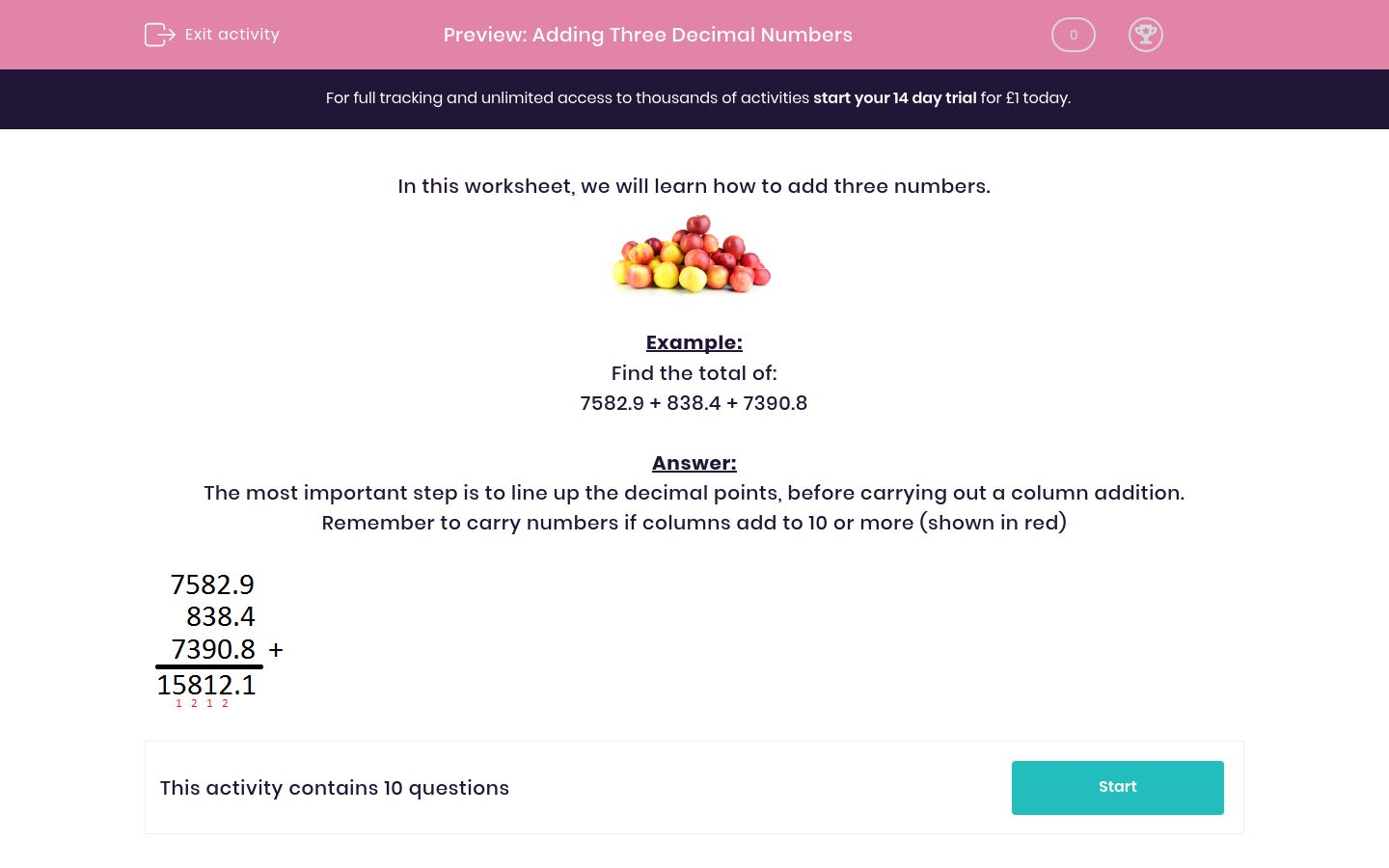### Affordable pricing from £10/month

In this worksheet, students practise adding together three decimal numbers - ensuring that the decimal points are in line before carrying out a column addition.Key stage:  KS 2

Curriculum topic:   Maths and Numerical Reasoning

Curriculum subtopic:   Decimals

Difficulty level:### QUESTION 1 of 10

In this worksheet, we will learn how to add three numbers.Example:

Find the total of:

7582.9 + 838.4 + 7390.8

The most important step is to line up the decimal points, before carrying out a column addition. Remember to carry numbers if columns add to 10 or more (shown in red)Find the total of

5006.1 + 3356.1 + 5492.8 = ___________

Find the total of

1432.8 + 1909.2 + 942.3 =  ___________

Find the total of

4643.4 + 8102.7 + 2279.2 =  ___________

Find the total of

4742.9 + 3201.9 + 3536.5 =  ___________

Find the total of

3838.2 + 8930.3 + 7174.1 =  ___________

Find the total of

3675.2 + 2449.5 + 357.5 =  ___________

Find the total of

5058.7 + 766.9 + 4003.7 =  ___________

Find the total of

6790.6 + 6708.4 + 4570.9 =  ___________

Find the total of

850.7 + 4148.1 + 2705.6 =  ___________

Find the total of

1451 + 8810.5 + 3085.3 =  ___________

• Question 1

Find the total of

5006.1 + 3356.1 + 5492.8 = ___________

13855
EDDIE SAYS
13855.0 is the same as 13855
• Question 2

Find the total of

1432.8 + 1909.2 + 942.3 =  ___________

4284.3
EDDIE SAYS
Line up the decimal points before adding
• Question 3

Find the total of

4643.4 + 8102.7 + 2279.2 =  ___________

15025.3
• Question 4

Find the total of

4742.9 + 3201.9 + 3536.5 =  ___________

11481.3
• Question 5

Find the total of

3838.2 + 8930.3 + 7174.1 =  ___________

19942.6
• Question 6

Find the total of

3675.2 + 2449.5 + 357.5 =  ___________

6482.2
• Question 7

Find the total of

5058.7 + 766.9 + 4003.7 =  ___________

9829.3
• Question 8

Find the total of

6790.6 + 6708.4 + 4570.9 =  ___________

18069.9
• Question 9

Find the total of

850.7 + 4148.1 + 2705.6 =  ___________

7704.4
• Question 10

Find the total of

1451 + 8810.5 + 3085.3 =  ___________

13346.8
EDDIE SAYS
1451 is the same as 1451.0
---- OR ----

Sign up for a £1 trial so you can track and measure your child's progress on this activity.

### What is EdPlace?

We're your National Curriculum aligned online education content provider helping each child succeed in English, maths and science from year 1 to GCSE. With an EdPlace account you’ll be able to track and measure progress, helping each child achieve their best. We build confidence and attainment by personalising each child’s learning at a level that suits them.

Get started